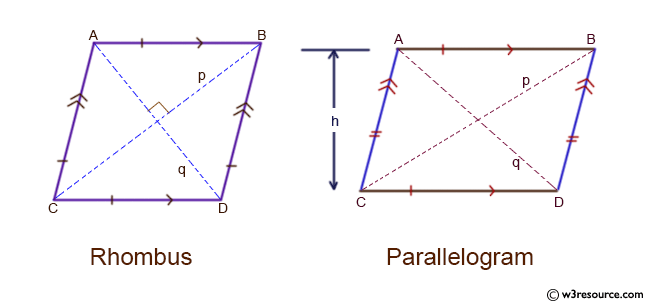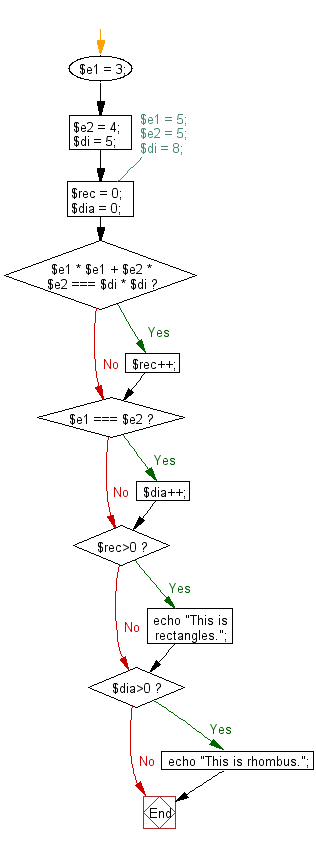﻿ PHP Exercise: Reads the two adjoined sides and the diagonal of a parallelogram and check whether the parallelogram is a rectangle or a rhombus - w3resource

# PHP Exercises: Reads the two adjoined sides and the diagonal of a parallelogram and check whether the parallelogram is a rectangle or a rhombus

## PHP: Exercise-62 with Solution

Write a PHP program which reads the two adjoined sides and the diagonal of a parallelogram and check whether the parallelogram is a rectangle or a rhombus.

According to Wikipedia-
parallelograms: In Euclidean geometry, a parallelogram is a simple (non-self-intersecting) quadrilateral with two pairs of parallel sides. The opposite or facing sides of a parallelogram are of equal length and the opposite angles of a parallelogram are of equal measure. rectangles: In Euclidean plane geometry, a rectangle is a quadrilateral with four right angles. It can also be defined as an equiangular quadrilateral, since equiangular means that all of its angles are equal (360°/4 = 90°). It can also be defined as a parallelogram containing a right angle. rhombus: In plane Euclidean geometry, a rhombus (plural rhombi or rhombuses) is a simple (non-self-intersecting) quadrilateral whose four sides all have the same length. Another name is equilateral quadrilateral, since equilateral means that all of its sides are equal in length. The rhombus is often called a diamond, after the diamonds suit in playing cards which resembles the projection of an octahedral diamond, or a lozenge, though the former sometimes refers specifically to a rhombus with a 60° angle (see Polyiamond), and the latter sometimes refers specifically to a rhombus with a 45° angle.

Input: Two adjoined sides and the diagonal.
1 ≤ ai, bi, ci ≤ 1000, ai + bi > ci

Pictorial Presentation:Sample Solution: -

PHP Code:

``````<?php
\$e1 = 3;
\$e2 = 4;
\$di = 5;
//\$e1 = 5;
//\$e2 = 5;
//\$di = 8;
\$rec = 0;
\$dia = 0;
if (\$e1 * \$e1 + \$e2 * \$e2 === \$di * \$di) {
\$rec++;
}
if (\$e1 === \$e2) {
\$dia++;
}
if (\$rec>0)
echo "This is rectangles.";
if (\$dia>0)
echo "This is rhombus.";
?>
```
```

Sample Input:
4,5,6
6,6,9

Sample Output:

`This is rectangles.`

Flowchart:PHP Code Editor:

Have another way to solve this solution? Contribute your code (and comments) through Disqus.

What is the difficulty level of this exercise?

Test your Programming skills with w3resource's quiz.

﻿

## PHP: Tips of the Day

Returns all elements in an array except for the first one

Example:

```<?php
function tips_tail(\$items)
{
return count(\$items) > 1 ? array_slice(\$items, 1) : \$items;
}

print_r(tips_tail([1, 5, 7]));
?>
```

Output:

```Array
(
 => 5
 => 7
)
```